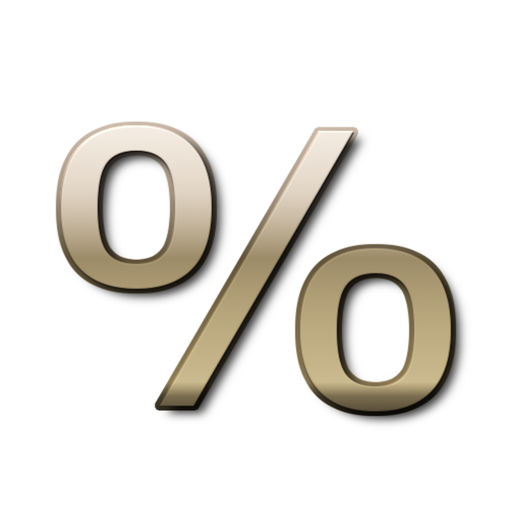Percentage Calculator 3 in one# Percentage Calculator 3 in one

## princely Ejikeme

Free
5.2

5 Ratings

Update Date

2013-12-31

Size

15.8 MB

### Screenshots for iPhone

iPhone
Percentage Calculator 3 in one Description
Percentage Calculator is a 3 in one hd app which calculates:
1 .Calculate Percents & Percentages
2. Percent Discounts (sale price)
3. Percent Markups (increase by)
4. Percent Margin
5. Percentage Difference (Change)
6.Percentage (what % of) i.e. x is what percentage of y

Percentage calculator is unique in a way that it calculates everything on the fly just by a touch of a button. it lets you find out any part of the equasion, for example in "5% of 40 is 2" you will enter any two of these three numbers and third one would be calculated for you. And that is what makes this percentage calculator stand out in the crowd.
Percentage calculator can be used in many real-life situations as calculator in
1. school (mathematics, statistics, algebra) calculator
3. shopping discounts calculator
4. cooking ingredients calculator
5. health (body mass index, fat percentage in food) calculator.
This app is a free version of percent calculator.
Percentage Calculator 3 in one 1.0.1 Update
2013-12-31 Version History
More
Price:
Free
Version:
1.0.1
Size:
15.8 MB
Genre: